# Disclinations

Disclinations are topological defects which share the same origin as dislocations from the Italian school (Volterra, Somigliana)

The formal process proposed by Volterra to create distorsions in a continuous medium (the so-called Volterra construction) serves as a definition and provide topological characteristics which lead to a classification of the various possible defects.

A cylinder of material oriented along the z-axis is cut along the yz-plane. The material on the two sides of the plane are displaced by d relative to each other and then glued together (with additional material inserted if needed).

In the most general case, the displacement d(r) is a combination of a translation b and of a rotation (w, t):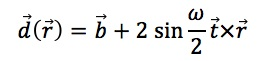It is however convenient to distinguish some simple cases: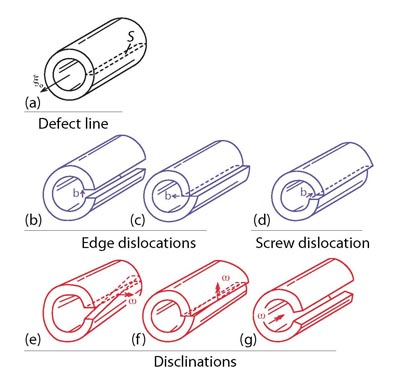1. The displacement d is a pure translation b: dislocations

A screw dislocation is created if b is along the a-axis (d), and two types of edge dislocations are created when b is in the xy-plane ((c) is the most common encountered case). The Volterra construction places no constraint on the displacement b. If the material is crystalline, the two sides will not glue together perfectly unless the Burgers vector b is a vector of the direct lattice. The direct lattice provides a quantization of the possible values of b. For energetic reasons, b will be chosen among the shortest direct lattice vectors.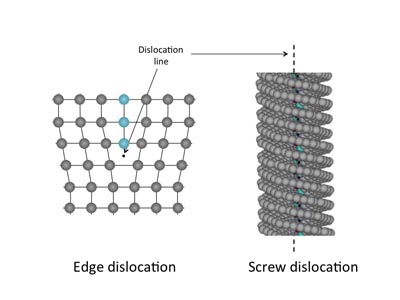2. There is another category of topological defects which occur when d results from a pure rotation. Following a suggestion of Frank, these defects have been called disclinations. They are energetically very costly and have been largely overlooked in solids. However, they have been described very early in liquid crystals (Friedel, 1922). Actually, it is from the observation of those defects that C. Friedel proposed its classification of liquid crystals into nematic, smectic and cholesteric phases. This illustrates the profound relationship between the symmetries of a medium and the nature of defects. Disclinations have also been used to describe deformations related to Bloch walls in ferromagnetic materials. Indeed, there is a strong analogy between disclinations in liquid crystals, defects in spin ordered ferromagnetic materials and vortices in hydrodynamics, superconductors and superfluids.

The Frank vector w represents for the disclinations what the Burgers vector is for dislocations. Similarly to dislocations, one considers the special cases when the Frank vector is parallel to the line vector (wedge disclination (g)) and when the Frank vector is perpendicular to the line (twist disclinations (e) & (f)).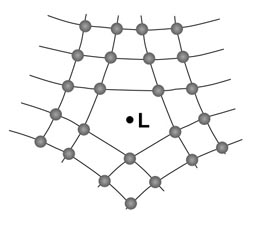A wedge disclination

The stress field induced by a wedge dislocation in a cylinder (inner radius rc, outer radius R) has been calculated by Timoshenko (1951):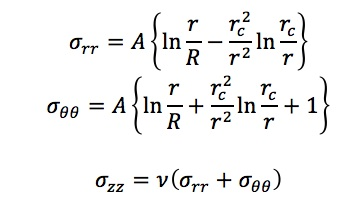where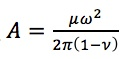Stresses and distortions increase with increasing distance r.

The elastic energy per unit length is: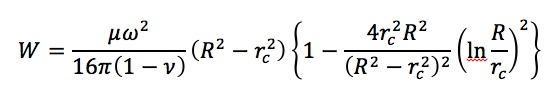This is of the same order of magnitude as an edge dislocation with a Burgers vector: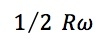In crystals, the elastic energy associated with disclinations is prohibitive, except in the form of self-screening configurations such as dipoles. Indeed, a wedge disclination dipole has a far-field elastic contribution similar to an edge dislocation with an equivalent Burgers vector: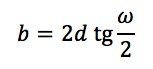where d is the distance between the two lines of the dipole measured perpendicular to the rotation vector.

3. When a translation and a rotation component are involved in the same time, one has a dispiration.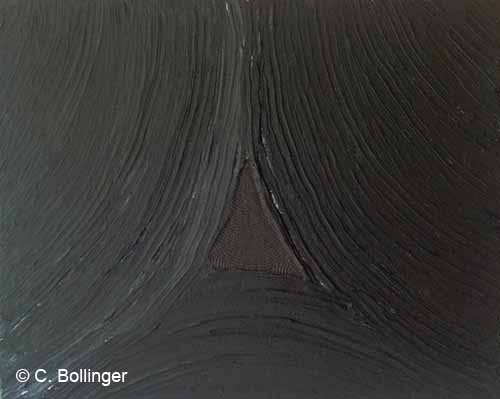References:

Friedel, G. (1922) Annls de Phys., 18, 273.

Timoshenko, s; (1951) Theory of elasticity. Mc Graw Hill, New York.

Volterra, V. (1907) Ann. Ecole Norm. Super. 24, 401.